# Texas Go Math Grade 1 Lesson 10.5 Answer Key Skip Count by Tens

Refer to our Texas Go Math Grade 1 Answer Key Pdf to score good marks in the exams. Test yourself by practicing the problems from Texas Go Math Grade 1 Lesson 10.5 Answer Key Skip Count by Tens.

## Texas Go Math Grade 1 Lesson 10.5 Answer Key Skip Count by Tens

Explore

Skip count by tens. Write to show how many crayons.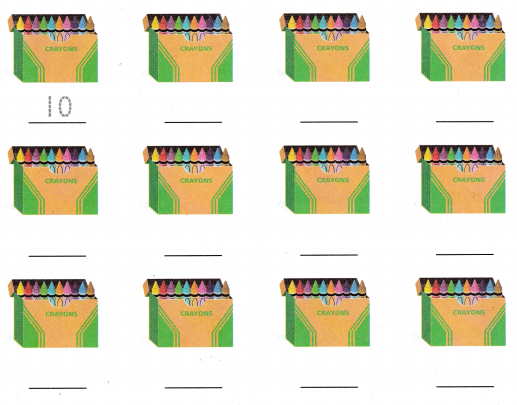When we skip by10 in between while counting the numbers, then it is said to skip counting by 10. Skip count by 10 is very easy and quick to do. See the below-given example to understand it better.
10+10=20
20+10=30
30+10=40 and so on…FOR THE TEACHER • Read the following problem. How many crayons would there be in 12 groups of 10 crayons?
The total number of groups=12
The number of crayons in each group=10
The total number of crayons in 12 groups=X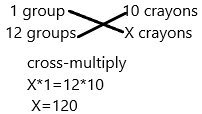Math Talk
Mathematical Processes

Explain When would it be useful to count tens?
Skip counting is often called counting by 2s or by 5s or by 10s or by 100s. Skip counting really helps with addition, subtraction, multiplication, and division. It is also used a lot for counting things – counting pairs of gloves, groups of five, money, boxes with 100 cans in each box – the list goes on!
How to skip count: To skip count you add the same number over and over. You can start at any number. When you count normally (like 1,2,3,4,5,6) you add 1 to get the next number. To count by 10s, you add 10 to get the next number.
Counting by tens: The two examples below show counting by tens. Look at the numbers. There is always a pattern.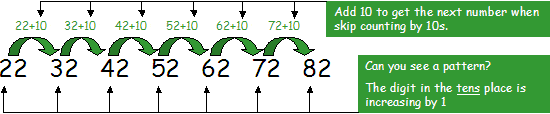Example 2:Model and Draw

Skip count the toes by tens to find how many.
How many are you counting each time?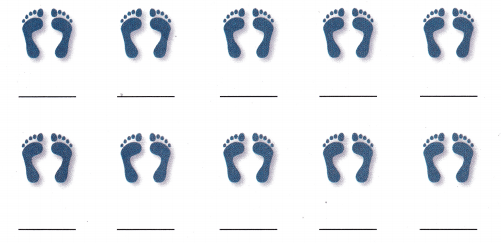Explanation:
1. Skip count in tens means to keep adding 10 to a number.
2. We can count up in tens from 10, adding ten each time.
3. We have 10, 20, 30, 40, 50, 60, 70, 80, 90, 100.
4. When skipping counting in tens, the number will all end in the same digit.
5. The digit in the tens column will increase by 1 each time.Share and Show

Skip count. Count the roses by tens. Write how many.

THINK: What is the best way to count these objects

Question 1.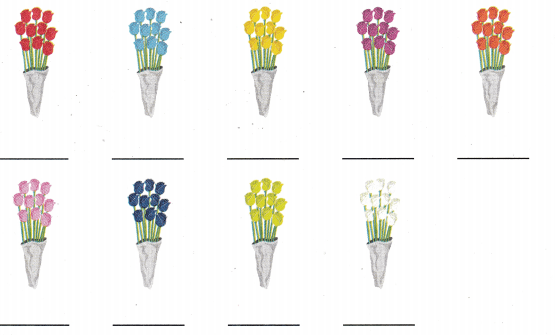Explanation:
1. Skip count in tens means to keep adding 10 to a number.
2. We can count up in tens from 10, adding ten each time.
3. We have 10, 20, 30, 40, 50, 60, 70, 80, 90.
4. When skipping counting in tens, the number will all end in the same digit.
5. The digit in the tens column will increase by 1 each time.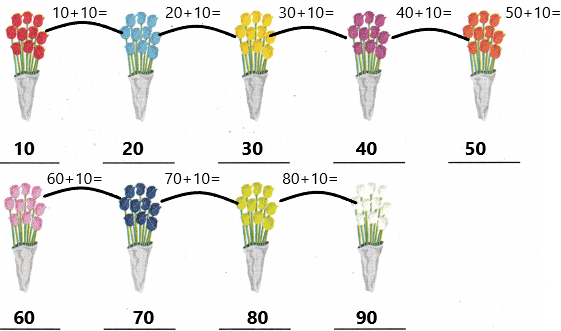Problem Solving

Skip count. Write how many.

Question 2.________ grapes
Explanation:
1. Skip count in tens means to keep adding 10 to a number.
2. We can count up in tens from 10, adding ten each time.
3. We have 10, 20, 30, 40, 50, 60, 70, 80, 90, 100, 110.
4. When skipping counting in tens, the number will all end in the same digit.
5. The digit in the tens column will increase by 1 each time.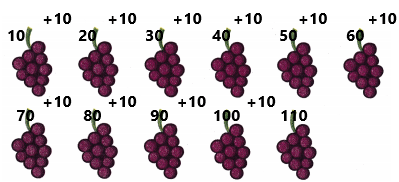Question 3._________ paintbrushes
Explanation:
1. Skip count in tens means to keep adding 10 to a number.
2. We can count up in tens from 10, adding ten each time.
3. We have 10, 20, 30, 40, 50, 60, 70, 80.
4. When skipping counting in tens, the number will all end in the same digit.
5. The digit in the tens column will increase by 1 each time.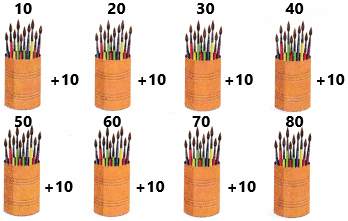Use skip counting by tens to solve. Write or draw to explain.

Question 4.
H.O.T. Vanessa swims 10 laps a day. How many laps does she swim in 5 days?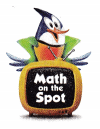________ laps
Explanation:
The number of laps she does in a day=10
The laps she swims in 5 days, we need to calculate now. Assume it as X.
Apply cross-multiplication.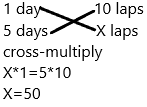Question 5.
H.O.T. Multi-Step There are 10 pencils in a packet. Justin has 6 packets. He buys 3 more packets. How many pencils does he have?
__________ pencils
Explanation:
The number of pencils in each packet=10
The number of packets Justin has=6
The number of packets he buys extra=3.
The total number of packets he has now=6+3=9.
Now calculate the total number of pencils in 9 packets=X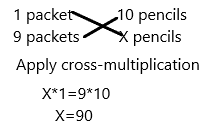Question 6.
Analyze Cody wants to make II trays of 10 tortillas. Skip count by tens. How many tortillas will he make?
(A) 21
(B) 110
(C) 100Explanation:
The number of tortillas Cody wants to make in 2 trays=10
The number of trays he wants to make=2
The number of tortillas in each tray=5
We need to use skip count by 10.In the first tray, he could make 50 tortillas and in the second tray, he could make 50 tortillas by using the skip count by 10 technique. I represented in the above diagram.
The total count of 2 trays of tortillas is 100.

Question 7.
Jaimie uses base ten blocks to show a number. Skip count by tens. What is the number shown?(A) 10
(B) 100
(C) 120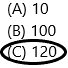Explanation:1. Skip count in tens means to keep adding 10 to a number.
2. We can count up in tens from 10, adding ten each time.
3. We have 10, 20, 30, 40, 50, 60, 70, 80, 90, 100, 110, 120.
4. When skipping counting in tens, the number will all end in the same digit.

Question 8.
Multi-Step There are 10 tables in a room. Each table has 10 seats. Six tables are full. No one is sitting at the other tables. How many seats are empty?
(A) 80
(B) 40
(C) 60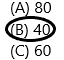Explanation:
The total number of tables in a room=10
The number of seats each table have=10
The total number of seats=10*10=100.
The number of tables full=6
This means 60 seats are already full. Now we need to find out the empty seats.
The number of empty seats=Total number of seats-filled seats
The number of seats=100-60=40.
Therefore, the empty seats are 40.

Question 9.
Texas Test Prep Which number comes after 110 when skip counting by tens?
(A) 120
(B) 80
(C) 100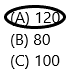Explanation:
1. Skip count in tens means to keep adding 10 to a number.
2. We can count up in tens from 10, adding ten each time.
3. We have 110, 120.
4. When skipping counting in tens, the number will all end in the same digit.
5. The digit in the tens column will increase by 1 each time.

TAKE HOME ACTIVITY • Have your child explain how he or she would count how many objects are in 12 pockets containing 10 objects each
The number of pockets=12
The number of objects in each pocket=10.
The total number of objects in 12 pockets=12*10=120.

### Texas Go Math Grade 1 Lesson 10.5 Homework and Practice Answer Key

Skip count. Count the fingers on the gloves by tens. Write how many.

Question 1.Explanation:
1. Skip count in tens means to keep adding 10 to a number.
2. We can count up in tens from 10, adding ten each time.
3. We have 10, 20, 30, 40, 50, 60, 70, 80, 90, 100, 110, 120.
4. When skipping counting in tens, the number will all end in the same digit.
5. The digit in the tens column will increase by 1 each time.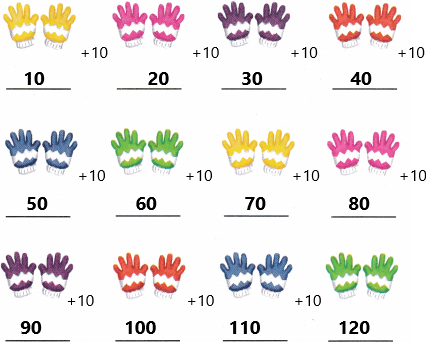Problem Solving

Use skip counting by tens to solve. Write or draw to explain.

Question 2.
Jason runs 10 laps around the track each day. How many laps does he run in 5 days?
________ laps
Explanation:
The number of laps he tracks each day=10
The number of laps he can make in 5 days=X
Apply cross-multiplicationLesson Check

Question 3.
Char’s mom is making cupcakes. She can make 10 cupcakes with one pan. She makes 12 pans of cupcakes. How many cupcakes did Char’s mom make?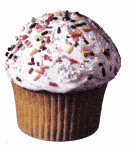(A) 120
(B) 100
(C) 18Explanation:
The number of pancakes she can make in one pan=10
The number of pans she has=12
The number of pancakes she made in 12 pans=12*10=120.

Question 4.
Paul counts his pennies. He draws a line for each group of 10 pennies he counts. How many pennies did Paul count?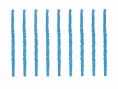(A) 80
(B) 100
(C) 90Explanation:
Count the number of lines he had drawn=9.
He draws a line for each group of pennies=10.
The total number of pennies the Paul count=9*10=90.

Question 5.
Multi-Step There are 10 party favors in each bag. Lonni buys 7 bags. Then he buys 3 more bags. How many favors did Lonni buy?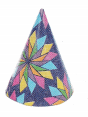(A) 70
(B) 100
(C) 30
Explanation:
The number of party favours in each bag=10
The number of bags he buy=7
The number of bags he buys extra=3
The total number of bags he has now=7+3=10.
The total number of party favours in 10 bags=X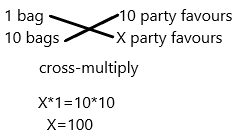Question 6.
Write the number that comes after 100 when skip counting by tens.